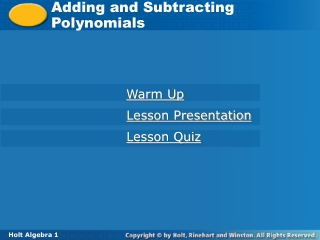DownloadDownload PresentationTélécharger la présentation- - - - - - - - - - - - - - - - - - - - - - - - - - - E N D - - - - - - - - - - - - - - - - - - - - - - - - - - -
Presentation Transcript

1. Adding and Subtracting Polynomials Warm Up Lesson Presentation Lesson Quiz Holt Algebra 1

2. Warm Up Simplify each expression by combining like terms. 1.4x + 2x 2. 3y + 7y 3. 8p – 5p 4. 5n + 6n2 Simplify each expression. 5. 3(x + 4) 6. –2(t + 3) 7. –1(x2 – 4x – 6) 6x 10y 3p not like terms 3x + 12 –2t – 6 –x2 + 4x + 6

3. Objective Add and subtract polynomials.

4. Just as you can perform operations on numbers, you can perform operations on polynomials. To add or subtract polynomials, combine like terms.

5. Example 1: Adding and Subtracting Monomials Add or Subtract.. A. 12p3 + 11p2 + 8p3 Identify like terms. 12p3 + 11p2 + 8p3 Rearrange terms so that like terms are together. 12p3 + 8p3 + 11p2 20p3 + 11p2 Combine like terms. B. 5x2 – 6 – 3x + 8

6. Example 1: Adding and Subtracting Monomials Add or Subtract.. C. t2 + 2s2– 4t2 –s2 D. 10m2n + 4m2n– 8m2n

7. Add or subtract. E. 2s2 + 3s2 + s F. 4z4– 8 + 16z4 + 2

8. Add or subtract. G. 2x8 + 7y8–x8–y8 H. 9b3c2 + 5b3c2– 13b3c2

9. 5x2+ 4x+1 + 2x2+ 5x+ 2 7x2+9x+3 Polynomials can be added in either vertical or horizontal form. In vertical form, align the like terms and add: In horizontal form, use the Associative and Commutative Properties to regroup and combine like terms. (5x2 + 4x + 1) + (2x2 + 5x+ 2) = (5x2 + 2x2 + 1) + (4x + 5x) + (1 + 2) = 7x2+ 9x+ 3

10. Example 2: Adding Polynomials Add. A. (4m2 + 5) + (m2 – m + 6) (4m2+ 5) + (m2– m + 6) Identify like terms. Group like terms together. (4m2+m2) + (–m)+(5 + 6) 5m2 – m + 11 Combine like terms. B. (10xy + x) + (–3xy + y)

11. Example 2C: Adding Polynomials Add. (6x2 – 4y) + (3x2 + 3y – 8x2 – 2y)

12. Check It Out! Example 2 Add (5a3 + 3a2 – 6a + 12a2) + (7a3–10a).

13. To subtract polynomials, remember that subtracting is the same as adding the opposite. To find the opposite of a polynomial, you must write the opposite of each term in the polynomial: –(2x3 – 3x + 7)= –2x3 + 3x– 7

14. Example 3A: Subtracting Polynomials Subtract. (x3 + 4y) – (2x3) Rewrite subtraction as addition of the opposite. (x3 + 4y) + (–2x3) (x3 + 4y) + (–2x3) Identify like terms. (x3– 2x3) + 4y Group like terms together. –x3 + 4y Combine like terms.

15. Example 3B: Subtracting Polynomials Subtract. (7m4 – 2m2) – (5m4 – 5m2 + 8)

16. Example 3C: Subtracting Polynomials Subtract. (–10x2 – 3x + 7) – (x2 – 9)

17. Example 3D: Subtracting Polynomials Subtract. (9q2 – 3q) – (q2 – 5)

18. Check It Out! Example 3 Subtract. (2x2 – 3x2 + 1) – (x2+ x + 1)

19. Example 4: Application A farmer must add the areas of two plots of land to determine the amount of seed to plant. The area of plot A can be represented by 3x2 + 7x – 5 and the area of plot B can be represented by 5x2 – 4x + 11. Write a polynomial that represents the total area of both plots of land.

20. Lesson Quiz: Part I Add or subtract. 1. 7m2 + 3m + 4m2 2. (r2 + s2) – (5r2 + 4s2) 3. (10pq + 3p) + (2pq – 5p + 6pq) 4. (14d2 – 8) + (6d2 – 2d +1) 11m2 + 3m (–4r2 – 3s2) 18pq – 2p 20d2 – 2d – 7 5. (2.5ab + 14b) – (–1.5ab + 4b) 4ab + 10b

21. Lesson Quiz: Part II 6. A painter must add the areas of two walls to determine the amount of paint needed. The area of the first wall is modeled by 4x2 + 12x + 9, and the area of the second wall is modeled by 36x2 – 12x + 1. Write a polynomial that represents the total area of the two walls. 40x2 + 10